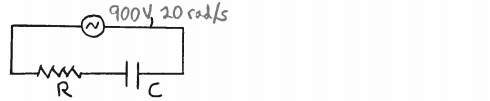# Problem: The circuit shown in the sketch has an ac voltage source, a resistor and a capacitor connected in series. There is no inductor. the ac voltage source has voltage amplitude 900 V and angular frequency 20 rad/s. The voltage amplitude across the capacitor is 500 V. The resistor has resistance R = 300 Ω.Does the source voltage lag or lead the current?

###### FREE Expert Solution
100% (478 ratings)
###### Problem Details

The circuit shown in the sketch has an ac voltage source, a resistor and a capacitor connected in series. There is no inductor. the ac voltage source has voltage amplitude 900 V and angular frequency 20 rad/s. The voltage amplitude across the capacitor is 500 V. The resistor has resistance R = 300 Ω.

Does the source voltage lag or lead the current?Frequently Asked Questions

What scientific concept do you need to know in order to solve this problem?

Our tutors have indicated that to solve this problem you will need to apply the Impedance in AC Circuits concept. You can view video lessons to learn Impedance in AC Circuits. Or if you need more Impedance in AC Circuits practice, you can also practice Impedance in AC Circuits practice problems.

How long does this problem take to solve?

Our expert Physics tutor, Juan took 1 minute and 18 seconds to solve this problem. You can follow their steps in the video explanation above.

What professor is this problem relevant for?

Based on our data, we think this problem is relevant for Professor Lyuksyutov's class at TAMU.